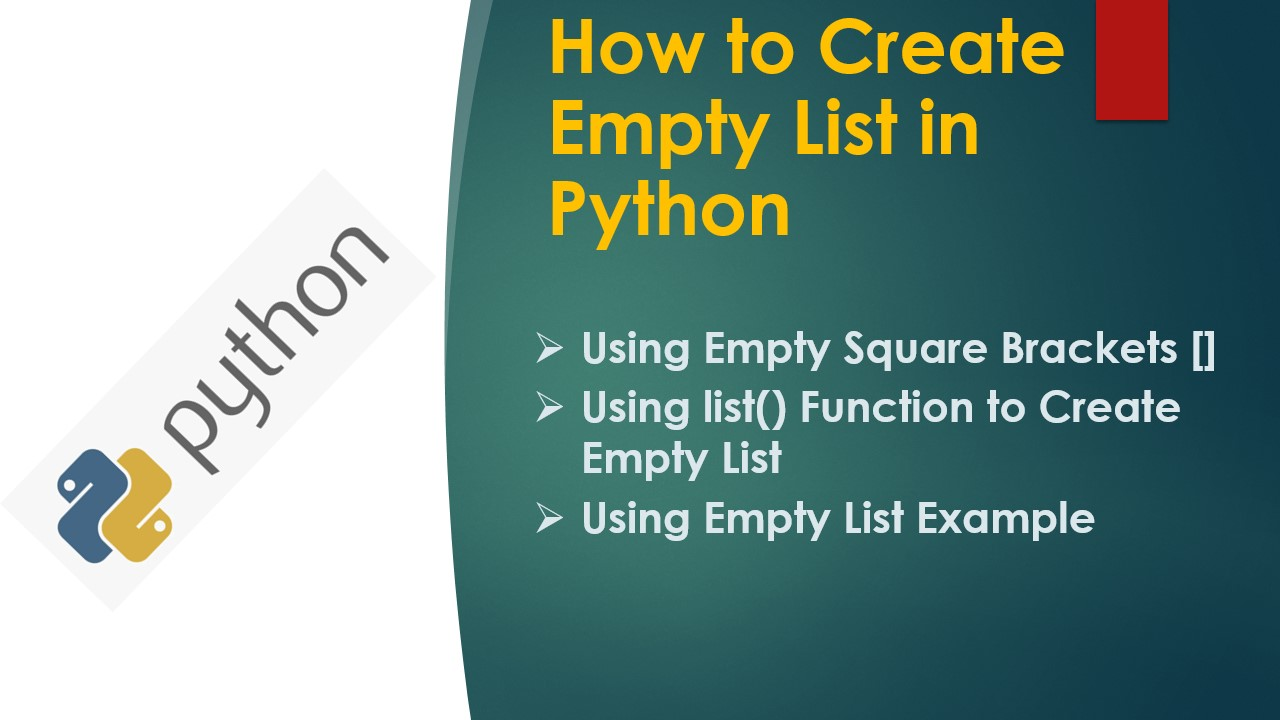# How to Create Empty List in Python

How to create an empty list in Python? You can create an empty list by using empty square brackets or using the list() function without any values.

Methods to create an empty list in Python

1. Create an empty list by using empty square brackets []
2. Create an empty list by using the list() function without arguments.

## What is an Empty List in Python?

A Python list is considered empty when it doesn’t have any elements, the length of the list is zero. The empty lists are considered Falsy meaning they evaluate to False when used with bool(). In the later sections, will see some examples.

## When you would use Empty List?

Empty lists are typically used when you wanted to incrementally create a list by using for loop. In this case, first, you initialize the list with empty and then iteratively add values to it in the `for loop`.

## 1. Quick Examples of Creating Empty List

Following are quick examples of creating an empty list.

``````
# Quick Examples of checking list is empty

# Example 1:
list1 = []

# Example 2:
list2 = list()
``````

## 2. Create Empty List Using Square Brackets []

Python lists are represented in square brackets and the elements are separated by a comma, so to create an empty list just use the empty square brackets [] without any elements. Here is an example.

``````
# Create empty list using []
list1 = []

# Print empty list
print(list1)

# Output:
# []
``````

When you print an empty list, it just prints empty square brackets. To check if the list is empty or not you can use the `len()` function which gives the number of elements in the list. Let’s check the size of the list.

``````
# Length of the list
print(len(list1))

# Output:
# 0
``````

The empty list is considered as `Falsy` and it evaluates to `Flase` when used with the `bool()`, if the list doesn’t have any elements then it returns `False` otherwise `True`. Here is an example.

``````
# Non empty list
list1 =[1,2,3,4]
print("List 1: ",list1)

# Empty list
list2 = []
print("List 2:", list2)

# Check if list is empty
print("Is List 1 Empty: ",not bool(list1))
print("Is List 2 Empty: ",not bool(list2))
``````

The above examples check if the python list is empty. Note that I used the `not` operator to invert the bool() result for more readability.

Here, I have considered the `list1` with some elements and `list2` with no elements. since I used the `not` along with bool() it reverses the result hence, the result shows True for list1 and False for list2.

## 3. Using list() Function to Create Empty List

Alternatively, you can use the Python list type constructor list() without any elements to create an empty list.

``````
# Create empty list using list()
list1 = list()

print(list1)

# Output:
# []
``````

## 4. Using Empty List Example

Let’s see the usage of an empty list with an example. Here, we created an empty list named list1, and inside for statement, we appended values to the empty list to create a new list.

``````
# Empty List reasltime usage
list1 = []
for x in range(10,30,5)
list1.append(x)
print(list1)

# Output:
# [10, 15, 20, 25]
``````

## Conclusion

In this article, you have learned how to create an empty list in Python by using the empty square brackets [] and list() function. And also learned the usage of an empty list with an example.

### Naveen

I am a Data Engineer with 20+ years of experience in transforming data into actionable insights. Over the years, I have honed my expertise in designing, implementing, and maintaining data pipelines with frameworks like Apache Spark, PySpark, Pandas, R, Hive and Machine Learning. My journey in the field of data engineering has been a continuous learning, innovation, and a strong commitment to data integrity. I have started this SparkByExamples.com to share my experiences with the data as I come across. You can learn more about me at LinkedIn